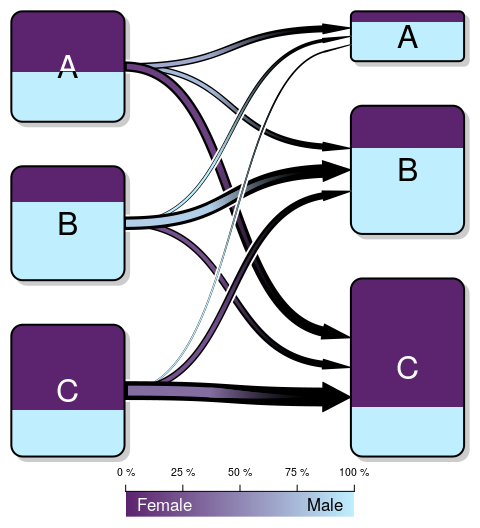# Introduction

The transitionPlot function aims at illustrating the transition between classes. The original intent was to show how self-administered the Charnley classification behaves before and after surgery. The plot is a fancier version than what can be achieved using packages such as diagram but at the cost of flexibility. The current function only allows to show a transition from one state to the next.

Note: The new Transition class will superseed the transitionPlot. See vignette("Introduction to the Transition-class") for more info.

# Generate some data

We will start by simulating some data similar to my article. Each observation has a sex and a Charnley class (A, B, or C). The transition is then dependent on both the sex and the Charnley class.

set.seed(1)
library(magrittr)
n <- 100
data <-
data.frame(
Sex = sample(c("Male", "Female"),
size = n,
replace = TRUE),
Charnley_class = sample(c("A", "B", "C"),
size = n,
replace = TRUE))
prob <- data.frame(
A = 1/3 +
(data$Sex == "Male") * .25 + (data$Sex != "Male") * -.25 +
(data$Charnley_class %in% "B") * -.5 + (data$Charnley_class %in% "C") * -2 ,
B = 1/3 +
(data$Sex == "Male") * .1 + (data$Sex != "Male") * -.05 +
(data$Charnley_class == "C") * -.5, C = 1/3 + (data$Sex == "Male") * -.25 +
(data$Sex != "Male") * .25) # Remove negative probabilities prob <- t(apply(prob, 1, function(x) { if (any(x < 0)){ x <- x - min(x) + .05 } x })) data$Charnley_class_after <-
apply(prob, 1, function(p)
sample(c("A", "B", "C"),
size = 1,
prob = p)) %>%
factor(levels = c("A", "B", "C"))

# Create the transition matrix that
# is the basis for the transition plot
trn_mtrx <-
with(data,
table(Charnley_class,
Charnley_class_after))

rm(prob)

# Basic use

The most simple use is to just supply the output from the table() call:

library(Gmisc)
transitionPlot(trn_mtrx)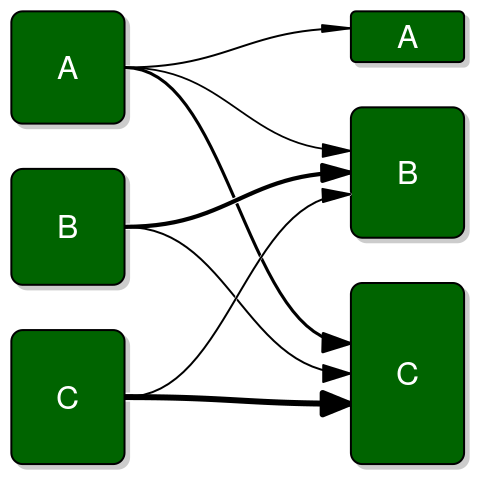The out of the box arrows are rather in my opinion not well suited and I have therefore developed a few alternatives:

transitionPlot(trn_mtrx,
type_of_arrow = "simple")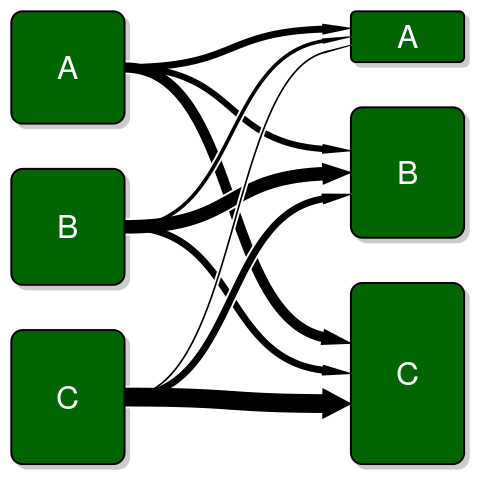And a fancy variant that I call gradient arrows as they have a colored gradient inside that fades into the arrow color:

transitionPlot(trn_mtrx,
type_of_arrow = "gradient")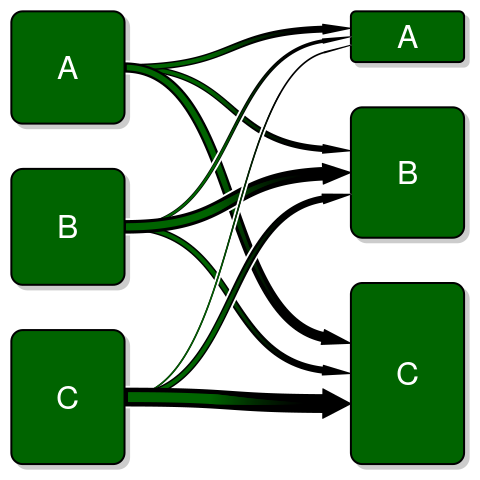# Labels and stuff

Adding title, box labels, and customizing box text can easily be done using the main, box_label, and box_txt. If you provide the box_txt a vector it assumes the same text for both left and right boxes but you can also have separate texts as in the example below:

output_perc <-
function(txt, n) sprintf("%s\n[%.0f%%]", txt, n)
box_txt <-
cbind(mapply(output_perc,
txt = c("A", "B", "C"),
n = prop.table(rowSums(trn_mtrx))*100),
mapply(output_perc,
txt = c("A", "B", "C"),
n = prop.table(colSums(trn_mtrx))*100))
transitionPlot(trn_mtrx,
main = "Charnley class changess",
box_label = c("Before", "After"),
box_txt = box_txt,
cex = 1.2,
type_of_arrow = "simple")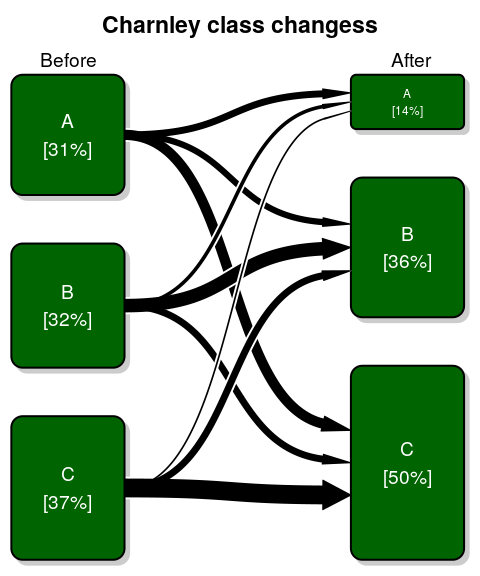# Using 3D matrices

We can enhance the data-ink ratio by adding a color split for the boxes. This works only for factors with 2 levels such as sex in our example.

trn_mtrx_3D <-
with(data,
table(Charnley_class,
Charnley_class_after,
Sex))

transitionPlot(trn_mtrx_3D,
fill_start_box = c("#5C246E", "#00688B"),
type_of_arrow = "simple")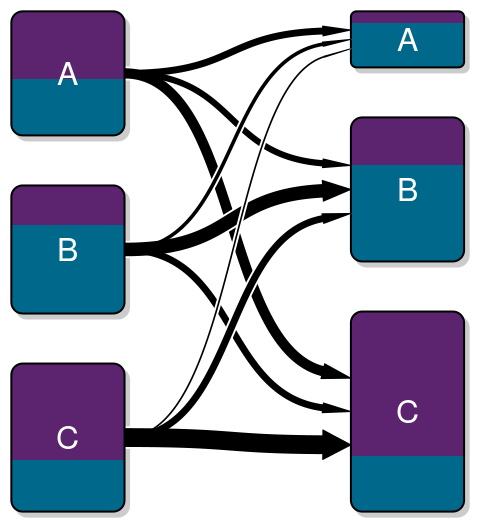The gradient arrow is most useful in this setting. It mixes the two colors according to the particular transition composition and adds an explaining gradient bar:

transitionPlot(trn_mtrx_3D,
fill_start_box = c("#5C246E", "#00688B"),
type_of_arrow = "gradient")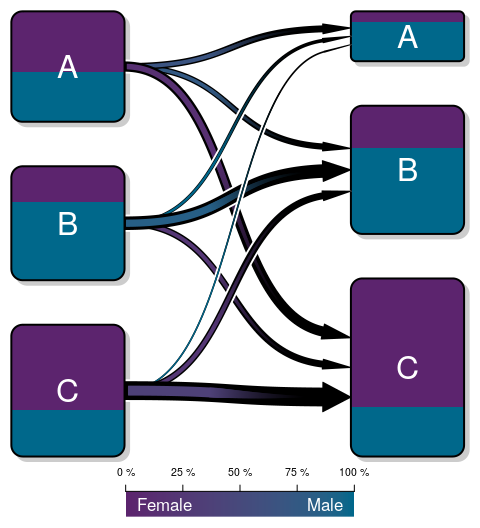Mixed text colors can be useful when one background color is brighter and white text becomes unreadable:

transitionPlot(trn_mtrx_3D,
txt_start_clr = c("white", "black"),
fill_start_box = c("#5C246E", "#BFEFFF"),
type_of_arrow = "gradient")